Yang, C., Xu, C., Su, J., He, W., and Gao, Z. (2021). "A new model based on principal component regression-random forest for analyzing and predicting the physical and mechanical properties of particleboard," BioResources 16(2), 2448-2471.

#### Abstract

The physical and mechanical properties are key indexes for determining the quality of particleboards. For this reason, a study on evaluating the physical and mechanical properties of particleboard via a new method has considerable value. Thus, a method based on principal component regression (PCR) analysis and random forest (RF) is proposed in this paper. First, the problems requiring resolution are described after analyzing the production process parameters as well as the physical and mechanical properties of particleboard. Then, an analysis and prediction models based on the PCR and RF method is established. On the basis of the PCR method, the key process parameters that affect various physical and mechanical properties are determined. Based on the RF method, the analysis and prediction model are built from the previously determined process parameters of the physical and mechanical properties. Finally, through experimental analysis, the effectiveness of the analysis and prediction models based on the PCR and RF method are verified. This work was able to determine the relationship between the process parameters and the physical and mechanical properties, which can help improve practical industrial manufacturing effectivity.

A New Model Based on Principal Component Regression-Random Forest for Analyzing and Predicting the Physical and Mechanical Properties of Particleboard

Cuiping Yang,a,b  Cong Xu,Jilai Su,Wei He,b,* and Zhenhua Gao a,*

The physical and mechanical properties are key indexes for determining the quality of particleboards. For this reason, a study on evaluating the physical and mechanical properties of particleboard via a new method has considerable value. Thus, a method based on principal component regression (PCR) analysis and random forest (RF) is proposed in this paper. First, the problems requiring resolution are described after analyzing the production process parameters as well as the physical and mechanical properties of particleboard. Then, an analysis and prediction models based on the PCR and RF method is established. On the basis of the PCR method, the key process parameters that affect various physical and mechanical properties are determined. Based on the RF method, the analysis and prediction model are built from the previously determined process parameters of the physical and mechanical properties. Finally, through experimental analysis, the effectiveness of the analysis and prediction models based on the PCR and RF method are verified. This work was able to determine the relationship between the process parameters and the physical and mechanical properties, which can help improve practical industrial manufacturing effectivity.

Keywords: Particleboard; Physical and mechanical properties; Process parameters; Property analyzing; Model prediction

Contact information: a: Northeast Forestry University, Harbin, Heilongjiang 150040 China; b: Harbin Normal University, Harbin, Heilongjiang 150025 China; c: Harbin Conservatory of Music, Harbin, Heilongjiang 150028 China; *Corresponding authors: 45604125@qq.com; 64282426@qq.com

INTRODUCTION

Particleboard refers to a type of wood-based panel, which is composed of pressed particles from branches, small-diameter wood, fast-growing wood, and sawdust with certain specifications (Kurt 2019; Kurt and Karayilmazlar 2019). Such materials are generally dried at a certain temperature and pressure, then mixed with glue, hardener, and a waterproofing agent. Particleboard is considered a renewable product, and it is generally used in many industries, e.g., for interior fitments (including furniture), construction, transportation, etc. (Hansted et al. 2019). Further studies and analyses regarding the physical properties and production process of particleboards would be beneficial for the improvement of their production processes and quality control. It would offer guidance in terms of improving the quality, production efficiency, as well as providing economic benefits (Hamzacebi 2016).

The physical and mechanical properties of particleboard are key indexes in determining the quality. Determining the physical and mechanical properties of particleboard during the production process is used to test and monitor the raw materials. This helps guarantee the quality of the final products (Huang et al. 2020). The physical and mechanical properties are crucial indexes for weighting the quality of the final product. Therefore, studying the impact of the process parameters on the final panel and the study of how to acquire physical and mechanical properties are valuable (Boon et al. 2013).

A traditional orthogonal test is typically used to study the influence of process parameters on the physical properties of the final panel, as demonstrated by Wu et al. (2016). Sugahara et al. (2019) manufactured particleboard using bagasse and eucalyptus slag pulp and tested its properties, i.e., the static bending strength, and internal bond strength. It was shown that the use of bagasse and eucalyptus slag in the production of high-density particleboard was feasible. Tupciauskas et al. (2019) proposed the use of non-formaldehyde biological-based adhesives in the particleboard production. Souza et al. (2018) tested the impact of each process parameter and their interactions on the physical and mechanical properties. The analysis was used to evaluate the feasibility of manufacturing particleboard with new adhesive types.

It is difficult to determine the level of actual parameters affecting the quality of particleboard in the production process because of the correlation of various process parameters (Owodunni et al. 2020). Orthogonal testing focuses on arranging the test scientifically in order to find the best combination of multiple factors. Nevertheless, scholars expect to find a method characterized by low trial numbers, short term, and high precision, as well as analyze the interaction among various factors. The response surface method meets these requirements. Islam et al. (2012) optimized the processing parameters of particleboard production via the response surface method. Nirdosha and Setunge (2006) used an experimental design and the response surface method to study the formula and process model made by waste wood obtained from hardwood sawmills. Then, an optimal set of processing parameters was determined using the physical and mechanical properties as well as economic considerations. Nazerian et al. (2015) determined the influence of four parameters on the static bending strength, internal bond strength, and water swelling. Nayeri et al. (2014) studied the influence of production parameters on the panel properties via the response surface method, and statistically analyzed the test results. Subsequently, the crucial parameters that had an impact on the panel properties were identified. However, it has been demonstrated that the response surface method is not suitable for industrial manufacturing. These tests require a large number of specimens and workers, which are time-consuming, labor-intensive, and costly.

The development of artificial intelligence technology has impacted the analysis process of physical and mechanical properties. It is considered a new testing method that requires less time, energy, and cost in comparison to traditional methods. Ozsahin (2013) used an artificial neural network model in order to predict the effects of certain production process parameters on the physical and mechanical properties of particleboard. Based on an artificial neural network, Esteban et al. (2009) determined the elastic modulus of wood by predicting its density, moisture content, and other parameters. Fernandez et al. (2008) resorted to artificial neural networks to predict the mechanical properties of particleboard. Therefore, it has been proposed that artificial neural networks are superior to any other multivariate regression model. Akyuz et al. (2017) used an artificial neural network to predict the formaldehyde emission of particleboards under different process parameter combinations. Valarmathi et al. (2020) analyzed various production conditions via an adaptive network-based fuzzy inference system and studied the influence of the process parameters on the surface roughness of particleboard. Considering that different process parameters have different effects on different physical and mechanical properties, unnecessary parameters will affect the prediction results when using all process parameters to predict the performance of particleboard (Kurt 2019). Therefore, this study uses a method that combines the principal component regression (PCR) method and the random forest (RF) method. First, the process parameters were analyzed via the PCR algorithm, and then the RF algorithm (a novel technique in the field of artificial intelligence) was used. This algorithm uses an integrated algorithm to further improve its accuracy and shows remarkable performance in terms of both classification and regression (Schubert et al. 2020). The PCR-RF method is used to analyze the process parameters and to predict the physical and mechanical properties of particleboard.

The innovations in this study are as follows: first, this paper will determine the key process parameters of different physical and mechanical properties through the analysis of actual enterprise production data; second, by establishing a predictive model, the mapping relation between process parameters and the physical and mechanical properties can be effectively realized, providing a basis for practical industrial manufacturing.

There are five parts to this paper. The first part is a general introduction. In the second part, the problems that will be solved are proposed and the process of solving these problems is defined. In the third part, the datasets used in this article are described, the physical performance analysis and the prediction model is built, and the implementation process is defined via the usage of the PCR method. The key process parameters of different physical and mechanical properties were analyzed via the usage of the RF method. In addition, a mapping model between the process parameters and the physical and mechanical properties was constructed. In the fourth part, the influencing factors and mapping relations of the different physical and mechanical parameters were determined via case analysis of eight different experiments, in order to prove the effectiveness of this method. In the last part, the research of this paper is summarized and discussed, and future research based off this study is defined.

Description of the Problems

The following three problems need to be resolved when establishing the analysis and prediction model.

Problem 1: Utilizing artificial intellectual technology as the basis, a new analysis and prediction model of the physical and mechanical properties of particleboard was established. In the actual production of particleboard, wood, sawdust, adhesive, and other raw materials ratio is fixed. Through the design of different process parameters, the physical and mechanical properties of the particleboard were transformed. Therefore, there are some mapping relationships between the physical and mechanical properties of particleboard and process parameters that should be analyzed. The relationships between the process parameters and the physical and mechanical properties of particleboard are described in Fig. 1.

Problem 2: Determining the key process parameters was required when the different physical and mechanical properties were analyzed. Different physical and mechanical properties were influenced by different process parameters by different degrees. Therefore, the key parameters were analyzed during the course of building the model that analyzed physical and mechanical properties. This process can be described as shown by Eq. 1,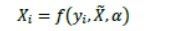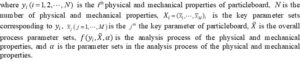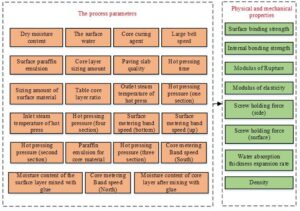Fig. 1. The process parameters and physical and mechanical properties of particleboard

Problem 3: Predicting the different physical and mechanical properties of particleboard requires the establishment of mapping relationships between the key process parameters and the physical and mechanical properties. In practical production, some corresponding relationships exist among the process parameters and the physical and mechanical properties, which contributes to predicting the real qualities of particleboard. Therefore, the relationship between the key process parameters and the physical and mechanical properties was analyzed while establishing the prediction model of the physical and mechanical properties. This analyzing process could be described as shown by Eq. 2,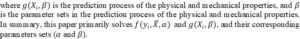EXPERIMENTAL

Materials

The data set used in this experiment is a real data set from a particleboard company in Shandong Province, China. The model number of these samples is 18E1P2, which is furniture type particleboard used in dry condition. The product specification is 18 mm, and the format is 1220 mm * 2440 mm. In this dataset, the wood raw materials of particleboard are all shavings, gum, paraffin emulsion, curing agent, and other chemical raw materials that have the same composition and ratio.

In terms of quantity, data were obtained twice a day at four-hour intervals. Based on the half year output of an enterprise in a production line, a total of 341 samples were collected. Each sample had 31 indicators, among which the first 23 indicators were process parameters, and the last 8 indicators were physical and mechanical properties. The process parameters were set by technicians according to experience. In the calculation of physical and mechanical properties, the particleboard was first to cut into 6 small pieces of 50 mm * 50 mm small particleboard pieces, and then the physical and mechanical properties of the six particleboards were tested, respectively. Finally, the average value was calculated as the physical and mechanical properties of the particleboard.

To verify the validity of the PCR-RF model, the data set was divided in a ratio of 2:8, with the first 272 samples in the data set as the training set and all samples as the test set. The prediction ability of the model was determined by comparing the output values of 69 untrained samples in the test set with the real physical and mechanical properties of the samples. By comparing the predicted results of the test set with the physical and mechanical properties of the real samples, the validity of the key process parameters obtained from the analysis was determined.

Prediction and Analyzing Model for Physical and Mechanical Properties Using the Principal Component Regression-Random Forest (PCR-RF) Method

The overall structure of the model

PCR and RF were combined to establish the model, which can be seen in Fig. 2.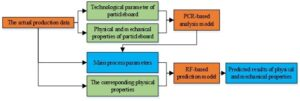Fig. 2. Particleboard analysis and prediction model

The primary components of the particleboard analysis and prediction model are defined as follows:

Step 1: the analysis model of the physical and mechanical properties was constructed. Stemming from actual production, the data of the technical parameters as well as the physical and mechanical properties were analyzed. The primary process parameters of each physical and mechanical property were determined via PCR.

Step 2: the prediction model of the physical and mechanical properties was constructed. The primary process parameters were used as input, and the physical and mechanical properties are used as output. The prediction results of the physical and mechanical properties were generated based on RF.

Analysis Model of the Physical and Mechanical Properties Using the Principal Component Regression (PCR) Method

Principal component regression is a method that analyzes and establishes a regression relationship with the principal component as the independent variable. This method is able to remove the multicollinearity problem and improve the final model (Liu et al. 2003; Lou and Zhao 2012; Liu et al. 2015). The analysis model is shown in Fig. 3.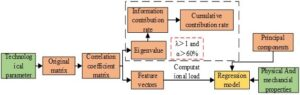Fig. 3. Analysis model of the physical and mechanical properties

Data standardization (step 1)

Suppose that there are  samples and each of them has  indicators. This process can be described by Eqs. 3 and 4,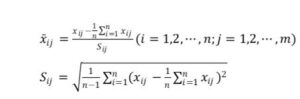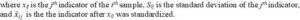Building matrix by calculating the correlation coefficient (step 2)

The process of calculating the correlation coefficient can be described by Eq. 5,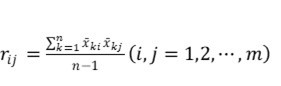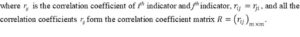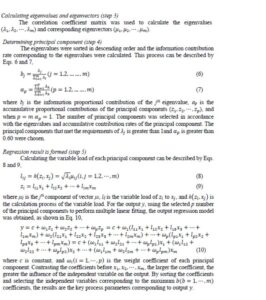Prediction Model of the Physical and Mechanical Properties Using the Random Forest (RF) Method

The RF algorithm, which is based on the Bagging algorithm, introduces an integrated learning method featuring random selection characteristics. With a decision tree as the base classification, a classifier is integrated through imitating a forest structure (Svetnik et al. 2003; Belgiu and Drăguţ 2016; Tyralis et al. 2019). The prediction model is shown in Fig. 4, and it is outlined below.Fig. 4. Prediction model using the random forest algorithm

First, the data set, which consists of the key process parameters determined via PCR analysis and correspondence with the physical and mechanical properties, was collected. The m samples were put from the data set each time to form a sampling set D, which is repeated T times to generate a total of  Dt(t=1,…,T) sample sets.

Next, the T decision trees were obtained by using sample set Dt to train the decision trees model Gt(X). When choosing the node for the decision tree model, the best trait in the whole node samples feature (k) is selected to make the dividing points of the left and right sub-tree (the split operation). When selecting a trait, the indicator impurity of the sub-branch is regarded as the base. The gene coefficient, information entropy, or information gain was regarded as the impurity measurement basis for classification problems.

The MSE (mean squared error) or RMSE (root mean square error) value was taken as the basis of the impurity valuation for regression problems. The MSE value was adopted in this experiment as the split base, which was calculated by Eq. 11 and Eq. 12,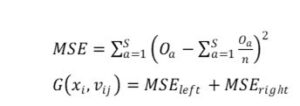where xi is the ith segmentation variables, vij is the segmentation value of the segmentation variables, n is the number of training samples after segmentation, Oa is the goal value of the  ath (a=1,…,S) sample after segmentation, MSEleft is the MSE of the segmented-left-subset, MSEright is the MSE value of the segmented-right-subset, and G(xi, vij) is the impurity of each node. The feature and value that minimize the sum of the MSE values of the left and right sets after splitting as the node were selected, i.e., the best segmentation feature and the best segmentation value. The above calculations were repeated for the remaining features until all training samples of all child nodes belonged to the same class or k = 0.

Finally, the regression results obtained via the arithmetic average obtained from the T decision trees were fed into the model to predict the results.

In the process of particleboard production, enterprises have different requirements for different physical and mechanical particleboard properties. Therefore, the key process parameters affected by different physical and mechanical properties were found, which is helpful for the effective adjustment of the process parameters during actual production.

When the material ratio and production equipment of the produced particleboards are fixed, the process parameters of the particleboard become the key factors affecting the physical and mechanical properties. Therefore, the physical and mechanical properties of particleboard can be predicted via the process parameters, which can effectively be utilized as a testing for particleboard.

RESULTS

This study aimed to create a predictive model to predict the physical and mechanical properties of particleboard in accordance with process parameters. During actual production, considering that the process parameters do not independently exist and there is a certain linear dependence, this will affect the final prediction results. Therefore, the combination of the principal component regression analysis algorithm and the random forest algorithm was selected to create the PCR-RF model. The specific steps are as follows:

Step 1: The 23 process parameters from the particleboard manufacturing process (as shown in Fig. 1) were formed into the original matrix, with a total of 341 sets of data and 23 indicators. In order to eliminate the dimensional influence between the indicators and ensure the comparability of the final errors, the samples were normalized to form a data set. This data set was analyzed via the principal component analysis method.

Step 2: The matrix was standardized to form a new matrix (Eqs. 3 and 4); the correlation coefficient was calculated among the indicators in the new matrix in order to form the correlation coefficient matrix (Eq. 5). Then, the eigenvalues and eigenvectors were calculated using the correlation coefficient matrix, and the information contribution rate of each eigenvalue was calculated (Eq. 6).

Step 3: The eigenvalues were sorted in descending order, and their cumulative information contribution rate was calculated (Eq. 7). The number of principal components was selected according to the following rules: The eigenvalue was greater than 1 and the cumulative information contribution rate was greater than 60%. In this experiment, the number of eigenvalues that were greater than 1 was 6, with a cumulative information contribution rate of 67.82%. It conformed to the rules mentioned above, so the number of principal components in this experiment was chosen as 6.

Step 4: With different physical and mechanical properties as the output, the 6 principal components were subjected to stepwise regression to obtain the results of the principal component regression analysis (Eqs. 8 and 9). According to the different physical and mechanical properties (Fig. 1), the corresponding linear fitting equations were built (Eq. 10). These equations used a logistic regression formula with the key process parameters as the original independent variables and the various physical and mechanical properties as the dependent variables. Then, the independent variables with considerable influence on the dependent variable were selected as the key process parameters, according to the independent variable coefficient size in the formula.

Step 5: In order to verify the validity of the model, for each physical and mechanical properties, its corresponding key process parameter was selected. They were used to form a matrix for each physical and mechanical property in this experiment, which was used as the data set the RF algorithm.

Step 6: By taking samples from the data set via replacement sampling to form a data subset, the decision tree was constructed (Eqs.11 and 12). Finally, the results of all the decision trees were averaged to obtain the prediction results of the random forest. The random forest parameters used in this experiment are shown in Table 1.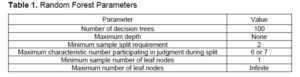Analysis and Prediction of the Internal Bonding Strength

The six principal components from the 23 process parameters were calculated via principal component analysis. Taking the internal bonding strength of the particleboard as the output, a stepwise regression was performed on the principal components, and the output regression model is shown in Eq. 13,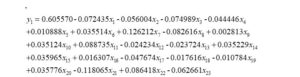where the definition of x1 ~ x23 and y1 ~ y8 in Eqs. 13 to 20 are shown in Table 2.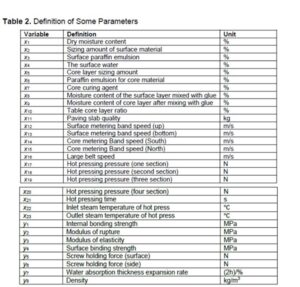With 272 specimens as the training set, all samples as the test set, and the internal bonding strength of particleboard and its corresponding seven process parameters as the index, the results of predicting the internal bonding strength of particleboard via the random forest algorithm are shown in Fig. 5.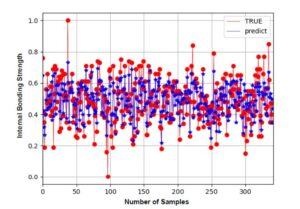Fig. 5. Prediction of the internal bonding strength of particleboard

Utilizing principal component regression analysis, different prediction methods were adopted to determine the physical and mechanical properties of particleboard. The results are shown in Table 3.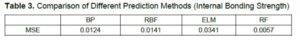Analysis and Prediction of the Modulus of Rupture

The six principal components from the 23 process parameters were calculated via principal component analysis. Taking the modulus of rupture of the particleboard as the output, a stepwise regression was performed on the principal components, and the output regression model is shown in Eq. 14,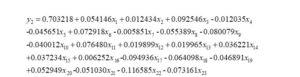With 272 samples as the training set, all samples as the test set, and the modulus of rupture of particleboard and its corresponding seven process parameters as the index, the results of predicting the modulus of rupture of particleboard via the random forest algorithm are shown in Fig. 6.Fig. 6.  Prediction of the modulus of rupture of particleboard

Utilizing principal component regression analysis, different prediction methods were adopted to determine the physical and mechanical properties of particleboard. The results are shown in Table 4.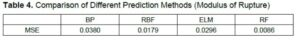Analysis and Prediction of the Modulus of Elasticity

The six principal components from the 23 process parameters were calculated via principal component analysis. Taking the modulus of elasticity of the particleboard as the output, a stepwise regression was performed on the principal components, and the output regression model is shown in Eq. 15,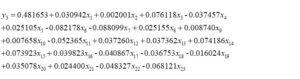With 272 samples as the training set, all samples as the test set, and the modulus of elasticity of particleboard and its corresponding seven process parameters as the index, the results of predicting the modulus of elasticity of particleboard via the random forest algorithm are shown in Fig. 7.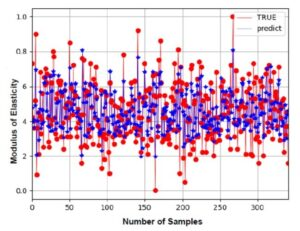Fig. 7. Prediction of the modulus of elasticity of particleboard

Utilizing principal component regression analysis, different prediction methods were adopted to determine the physical and mechanical properties of particleboard. The results are shown in Table 5.Analysis and Prediction of the Surface Binding Strength

The six principal components from the 23 process parameters were calculated via principal component analysis. Taking the surface binding strength of the particleboard as the output, a stepwise regression was performed on the principal components, and the output regression model is shown in Eq. 16,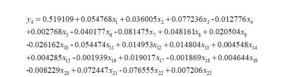With 272 samples as the training set, all samples as the test set, and the surface binding strength of particleboard and its corresponding seven process parameters as the index, the results of predicting the surface binding strength of particleboard via the random forest algorithm are shown in Fig. 8.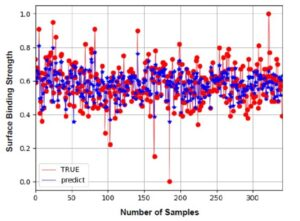Fig. 8. Prediction of the surface binding strength of particleboard

Utilizing principal component regression analysis, different prediction methods were adopted to determine the physical and mechanical properties of particleboard. The results are shown in Table 6.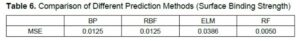Analysis and Prediction of the Screw Holding Force (Surface)

The six principal components from the 23 process parameters were calculated via principal component analysis. Taking the screw holding force (surface) of the particleboard as the output, a stepwise regression was performed on the principal components, and the output regression model is shown in Eq. 17,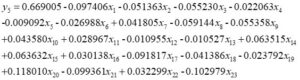With 272 samples as the training set, all samples as the test set, and the screw holding force (surface) of particleboard and its corresponding seven process parameters as the index, the results of predicting the screw holding force (surface) of particleboard via the random forest algorithm are shown in Fig. 9.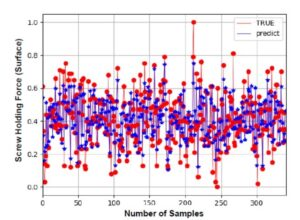Fig. 9. Prediction of the screw holding force (surface) of particleboard

Utilizing principal component regression analysis, different prediction methods were adopted to determine the physical and mechanical properties of particleboard. The results are shown in Table 7.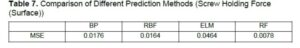Analysis and Prediction of the Screw Holding Force (Side)

The six principal components from the 23 process parameters were calculated via principal component analysis. Taking the screw holding force (side) of the particleboard as the output, a stepwise regression was performed on the principal components, and the output regression model is shown in Eq. 18,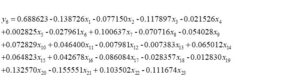With 272 samples as the training set, all samples as the test set, and the screw holding force (side) of particleboard and its corresponding seven process parameters as the index, the results of predicting the screw holding force (side) of particleboard via the random forest algorithm are shown in Fig. 10.Fig. 10. Prediction of the screw holding force (side) of particleboard

Utilizing principal component regression analysis, different prediction methods were adopted to determine the physical and mechanical properties of particleboard. The results are shown in Table 8.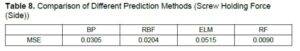Analysis and Prediction of the Water Absorption Thickness Expansion Rate

The six principal components from the 23 process parameters were calculated via principal component analysis. Taking the water absorption thickness expansion rate of the particleboard as the output, a stepwise regression was performed on the principal components, and the output regression model is shown in Eq. 19,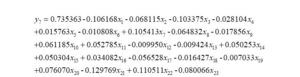With 272 samples as the training set, all samples as the test set, and the water absorption thickness expansion rate of particleboard and its corresponding seven process parameters as the index, the results of predicting the water absorption thickness expansion rate of particleboard via the random forest algorithm are shown in Fig. 11.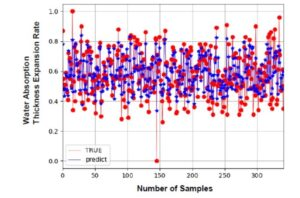Fig. 11. Prediction of the water absorption thickness expansion rate of particleboard

The random forest algorithm was utilized as the prediction method to analyze the original data set with different regression analysis methods. The study applied different prediction methods to predict the physical and mechanical properties of particleboard, and the results shown in Table 9.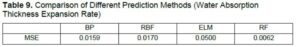Analysis and Prediction of Density

The six principal components from the 23 process parameters were calculated via principal component analysis. Taking the density of the particleboard as the output, a stepwise regression was performed on the principal components, and the output regression model is shown in Eq. 20,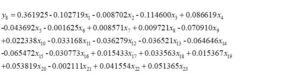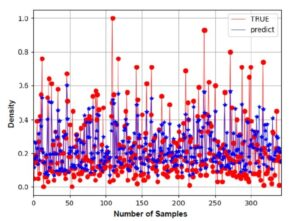Fig. 12. Prediction of Density of particleboard

With 242 samples as the training set, all samples as the test set, and the density of particleboard and its corresponding seven process parameters as the index, the results of predicting the density of particleboard via the random forest algorithm are shown in Fig. 11.

The random forest algorithm was utilized as the prediction method to analyze the original data set with different regression analysis methods. The study applied different prediction methods to predict the physical and mechanical properties of particleboard, and the results shown in Table 10.For Eq. 13-Eq. 20, the results of regression ability analysis are shown in Table 11.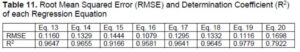DISCUSSION

Through Eqs. 13 to 20, the key process parameters that affect different physical and mechanical properties were obtained in this paper, as shown in Table 12. Some process parameters have positive effects on the final physical and mechanical properties, while some process parameters had negative effects. In addition, each physical and mechanical property had certain process parameters that had the most significant impact on it.

As shown in Tables 3 to 10, different machine learning methods to predict the results of the MSE value yielded different results. The RF effect on the physical and mechanical properties of particleboard prediction was the best, followed by BP and RBF. The effect of the ELM was the worst. It may be that the RF adopted the thought of integrated study, can, by using the method of integration of multiple decision trees to reduce the generalization error, get the best results. Other methods, as derivative methods of neural networks, may require more samples to obtain more accurate prediction results.

Take the internal bonding strength of particleboard as an example, as shown in Fig. 5. The physical and mechanical properties of the original data are basically consistent with the physical and mechanical properties predicted by the PCR-RF model, which shows the effectiveness of the key process parameters analyzed by the model.

In this experiment, last 69 samples in the data set were not trained. These 69 samples were input into the model, and the prediction ability of the model could be verified by comparing the output values of the model with the real physical and mechanical properties of the samples. As shown in Fig. 13, the physical and mechanical properties of particleboard predicted by the PCR-RF model are roughly similar to those of the actual particleboard, which proves the predictive ability of the model.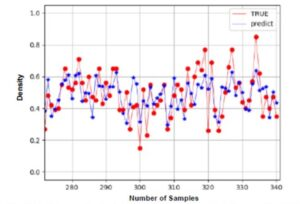Fig. 13. Prediction results of the last 69 samples of the internal bonding strength in Particleboard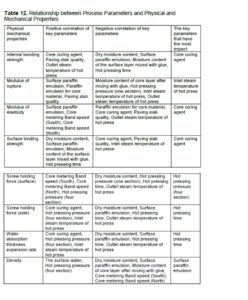Future Opportunities

In future research, the RF structure and parameters will be optimized, and the prediction accuracy of the model will be improved. The application of deep learning technology in particleboard production will be explored. More analysis methods will be used to analyze the key process parameters.

CONCLUSIONS

1. This paper has established that the physical and mechanical properties of particleboard can be predicted by artificial intelligence based on the process parameters. Among the common machine learning methods, the prediction effect of random forest is the best.
2. The PCR method can effectively analyze the process parameters that affect the performance of particleboard, so as to find the corresponding production stage, further improve the quality of particleboard, and provide direction for actual production.
3. The PCR-RF method proposed in this paper combines particleboard with artificial intelligence technology. This method not only can reduce the cost, time, and energy of enterprises in measuring the physical and mechanical properties of particleboard, but also find the key process parameters corresponding to the physical and mechanical properties of particleboard, so as to help the production of particleboard.

ACKNOWLEDGMENTS

This work was supported in part by the Fundamental Research Funds for the Central Universities (Grant No. 2572018CP01), in part by the Natural Science Foundation of Heilongjiang Province of China (Grant No. F2018023), and in part by the Ph.D. research start-up Foundation of Harbin Normal University (Grant No. XKB201905), and in part by the Natural Science Foundation of School of Computer Science and Information Engineering, Harbin Normal University (Grant no. JKYKYZ202102).

REFERENCES CITED

Akyüz, İ., Özşahin, Ş., Tiryaki, S., and Aydin, A. (2017). “An application of artificial neural networks for modeling formaldehyde emission based on process parameters in particleboard manufacturing process,” Clean Technologies and Environmental Policy 19(5), 1449-1458. DOI: 10.1007/s10098-017-1342-0

Belgiu, M., and Drăguţ, L. (2016). “Random forest in remote sensing: A review of applications and future directions,” ISPRS Journal of Photogrammetry and Remote Sensing 114, 24-31. DOI: 10.1016/j.isprsjprs.2016.01.011

Boon, J. G., Hashim, R., Sulaiman, O., Hiziroglu, S., Sugimoto, T., and Sato, M. (2013).  “Influence of processing parameters on some properties of oil palm trunk binderless particleboard,” European Journal of Wood and Wood Products 71(5), 583-589. DOI: 10.1007/s00107-013-0712-5

Esteban, L. G., Fernandez, F. G., and de Palacios, P. (2009). “MOE prediction in Abies pinsapo Boiss. timber: Application of an artificial neural network using non-destructive testing,” Computers & Structures 87(21-22), 1360-1365. DOI: 10.1016/j.compstruc.2009.08.010

Fernández, F. G., Esteban, L. G., de Palacios, P., Navarro, N., and Conde, M. (2008). “Prediction of standard particleboard mechanical properties utilizing an artificial neural network and subsequent comparison with a multivariate regression model,” Forest Systems 17(2), 178-187. DOI: 10.5424/srf/2008172-01033

Hamzaçebi, C. (2016). “Optimization of process parameters in oriented strand board manufacturing by Taguchi method,” Bioresources 11(3), 5987-5993. DOI: 10.15376/biores.11.3.5987-5993

Hansted, F. A. S., Hansted, A. L. S., Padilla, E. R. D., Caraschi, J. C., Goveia, D., and de Campos, C. I. (2019). “The use of nanocellulose in the production of medium density particleboard panels and the modification of its physical properties,” BioResources 14(3), 5071-5079. DOI: 10.15376/biores.14.3.5071-5079

Huang, H.-K., Hsu, C.-H., Hsu, P.-K., Cho, Y.-M., Chou, T.-H., and Cheng, Y.-S. (2020). “Preparation and evaluation of particleboard from insect rearing residue and rice husks using starch/citric acid mixture as a natural binder,” Biomass Conversion and Biorefinery DOI: 10.1007/s13399-020-00994-6

Islam, A., Alam, R., and Hannan, O. (2012). “Multiresponse optimization based on statistical response surface methodology and desirability function for the production of particleboard,” Composites Part B: Engineering 43(3), 861-868. DOI: 10.1016/j.compositesb.2011.11.033

Kurt, R. (2019). “Determination of the most appropriate statistical method for estimating the production values of medium density fiberboard,” BioResources 14(3), 6186-6202. DOI: 10.15376/biores.14.3.6186-6202.

Kurt, R., and Karayilmazlar, S. (2019). “Estimating modulus of elasticity (MOE) of particleboards using artificial neural networks to reduce quality measurements and costs,” Drvna Industrija 70(3), 257-263. DOI: 10.5552/drvind.2019.1840.

Liu, R. X., Kuang, J., Gong, Q.,  and  Hou, X. L. (2003). “Principal component regression analysis with SPSS,” Computer Methods and Programs in Biomedicine 71(2), 141-147. DOI: 10.1016/S0169-2607(02)00058-5

Liu, Y., Hu, W., Guo, X.-X., Wang, X.-C., Sun,  S.-Q., and  Xu, C.-H. (2015). “Rapid discrimination of three marine fish surimi by Tri-step infrared spectroscopy combined with principle component regression,” Spectrochimica Acta Part A: Molecular and Biomolecular Spectroscopy 149, 516-522. DOI: 10.1016/j.saa.2015.04.116

Lou, I., and Zhao, Y. (2012). “Sludge bulking prediction using principle component regression and artificial neural network,” Mathematical Problems in Engineering 2012, 295-308. DOI: 10.1155/2012/237693

Nayeri, M. D., Tahir, P., Jawaid, M., Harun, J., Abdullah, L. C., Bakar, E. S., Keshani, S., and Namvar, F. (2014). “Effect of resin content and pressure on the performance properties of rubberwood-kenaf composite board pane,” Fibers and Polymers 15(6), 1263-1269. DOI: 10.1007/s12221-014-1263-z

Nazerian, M., Dalirzadeh, A., and Farrokhpayam, S. R. (2015). “Use of almond shell powder in modification of the physical and mechanical properties of medium density fiberboard,” BioResources 10(1), 169-181. DOI: 10.15376/biores.10.1.169-181

Nirdosha, G., and Setunge, S. (2006). “Formulation and process modeling of particleboard production using hardwood saw mill wastes using experimental design,” Composite Structures 75(1-4), 520-523. DOI: 10.1016/j.compstruct.2006.04.044

Owodunni, A. A., Lamaming, J., Hashim, R., Taiwo, O. F. A., Hussin, M. H., Kassim, M. H. M., Bustami, Y., Sulaiman, O., Amini, M. H. M., and Hiziroglu, S. (2020). “Adhesive application on particleboard from natural fibers: A review,” Polymer Composites 41(11), 4448-4460. DOI:10.1002/pc.25749

Ozsahin, S. (2013). “Optimization of process parameters in oriented strand board manufacturing with artificial neural network analysis,” European Journal of Wood and Wood Products 71(6), 769-777. DOI: 10.1007/s00107-013-0737-9

Schubert, M., Luković, M., and Christen, H. (2020). “Prediction of mechanical properties of wood fiber insulation boards as a function of machine and process parameters by random forest,” Wood Science and Technology 54(3), 703-713. DOI: 10.1007/s00226-020-01184-3

Souza, A. M., Nascimento, M. F., Almeida, D. H., Silva, D. A. L., Almeida, T. H., Christoforo, A. L., and Lahr, F. A. R. (2018). “Wood-based composite made of wood waste and epoxy based ink-waste as adhesive: A cleaner production alternative,” Journal of Cleaner Production 193, 549-562. DOI: 10.1016/j.jclepro.2018.05.087

Sugahara, E. S., da Silva, S. A. M., Buzo, A. L. S. C., de Campos, C. I.,  Morales, E. A. M., Ferreira, B. S., Azambuja, M. d. A., Lahr, F. A. R., and Christoforo, A. L. (2019). “High-density particleboard made from agro-industrial waste and different adhesives,” BioResources 14(3), 5162-5170. DOI: 10.15376/biores.14.3.5162-5170

Svetnik, V., Liaw, A., Tong, C., Culberson, J. C., Sheridan, R. P., and Feuston, B. P. (2003). “Random forest: A classification and regression tool for compound classification and QSAR modeling,” Journal of Chemical Information and Computer Sciences 43(6), 1947-1958. DOI: 10.1021/ci034160g

Tupciauskas, R., Rizhikovs, J., Grinins, J., Paze, A., Andzs, M., Brazdausks, P., Puke, M., and Plavniece, A. (2019). “Investigation of suberinic acids-bonded particleboard,” European Polymer Journal 113, 176-182. DOI: 10.1016/j.eurpolymj.2019.01.061

Tyralis, H., Papacharalampous, G., and Langousis, A. (2019). “A brief review of random forests for water scientists and practitioners and their recent history in water resources,” Water 1(5), 1-37. DOI: 10.3390/w11050910

Valarmathi, T. N., Palanikumar, K., Sekar, S., and Latha, B. (2020). “Investigation of the effect of process parameters on surface roughness in drilling of particleboard composite panels using adaptive neuro fuzzy inference system,” Materials and Manufacturing Processes 35(4), 469-477. DOI: 10.1080/10426914.2020.1711931

Wu, X., Wang, X., Zhao, Z., and Liu, J. (2016). “Basic physicochemical properties and the optimization of particleboard production process of Salix discolor timber,” Journal of Northwest Forestry University 31(4), 259-264.

Article submitted: November 29, 2020: Peer review completed: January 16, 2021; Revised version received and accepted: February 6, 2021; Published: February 9, 2021.

DOI: 10.15376/biores.16.2.2448-2471